15 篇文章 14 订阅

第一部分  入门教程

机器学习简介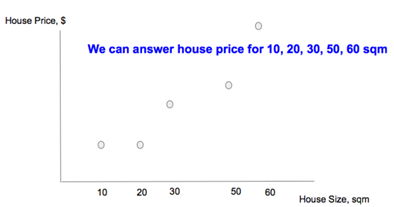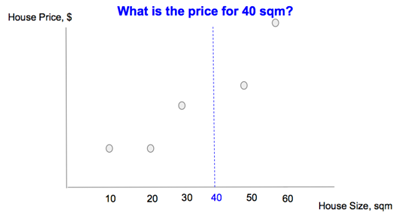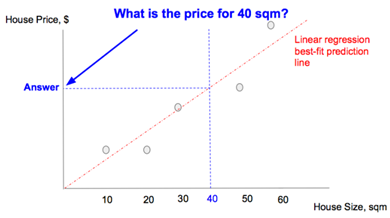步骤一：选择一个模型

1.模型种类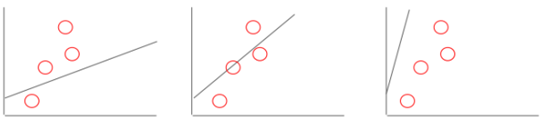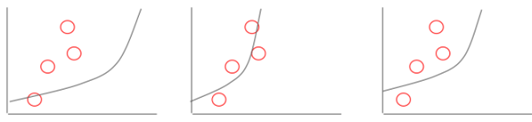2.成本函数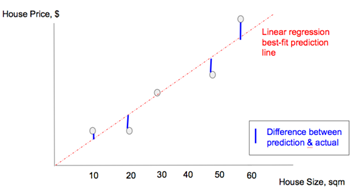3.线性模型简介

y = W.x + b
Where:
x: house size, in sqm
y: predicted house price, in $为了调整模型来更好地拟合数据点，我们可以这样做： 1）调整 W 来改变线性模型的梯度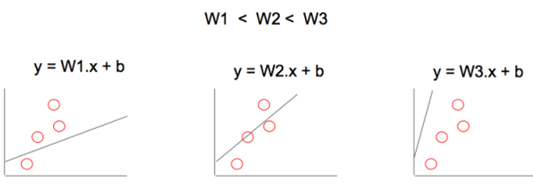2）调整 b 来改变线性模型的位置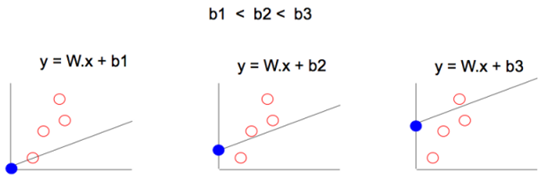通过使用许多个 W、b 的值，最终我们可以找到一个最佳拟合线性模型，能够将成本函数降到最小。 除了随机尝试不同的值，有没有一个更好的方法来快速找到 W、b 的值？ 4.梯度下降 如果你试图从山上下降到最低点，很多方式下的下降趋势并不明显！ 其最佳方式是执行梯度下降： • 在当前位置以最陡的下降梯度确定方向 • 在该方向上采取步长 X • 重复 & 刷新；这就是训练过程 最小化成本函数是类似的，因为成本函数就像是起伏的山，我们想要找到其中的最低点，我们可以通过梯度下降类似地实现。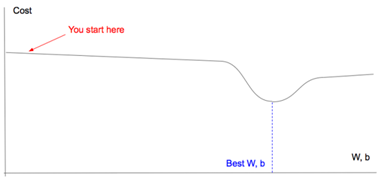现在我们有了线性模型、成本函数和梯度下降的概念，可以开始使用 TensorFlow 了。 步骤二：在TensorFlow 中建立模型 1.TensorFlow 中的线性模型 TensorFlow 的2个基本组件是： 1）占位符（Placeholder）：表示执行梯度下降时将实际数据值输入到模型中的一个入口点。例如房子面积 (x) 和房价 (y_)。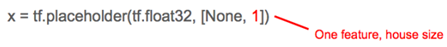2）变量：表示我们试图寻找的能够使成本函数降到最小的「good」值的变量，例如 W 和 b。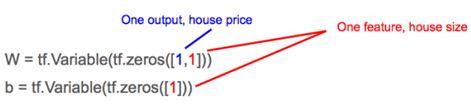然后 TensorFlow 中的线性模型 (y = W.x + b) 就是：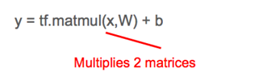2.TensorFlow 中的成本函数 与将数据点的实际房价 (y_) 输入模型类似，我们创建一个占位符。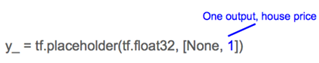成本函数的最小方差就是：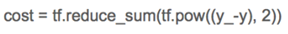3.数据 由于没有房价(y_) 和房子面积 (x) 的实际数据点，我们就生成它们。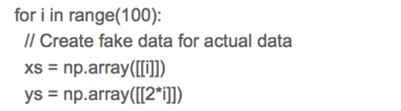简单起见，我们将房价 (ys) 设置成永远是房子面积 (xs) 的 2 倍。 4.梯度下降 有了线性模型、成本函数和数据，我们就可以开始执行梯度下降从而最小化代价函数，以获得 W、b 的「good」值。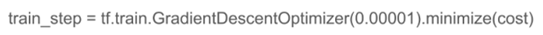0.00001 是我们每次进行训练时在最陡的梯度方向上所采取的「步」长；它也被称作学习率（learning rate）。 步骤三：训练模型 训练包含以预先确定好的次数执行梯度下降，或者是直到成本函数低于某个预先确定的临界值为止。 1.TensorFlow 的怪异 所有变量都需要在训练开始时进行初始化，否则它们可能会带有之前执行过程中的残余值。 2.TensorFlow 会话 虽然 TensorFlow 是一个 Python 库，Python 是一种解释性的语言，但是默认情况下不把 TensorFlow 运算用作解释性能的原因，因此不执行上面的 init 。相反 TensorFlow 是在一个会话中进行；创建一个会话 (sess) 然后使用 sess.run() 去执行。 类似地我们在一个循环中调用 withinsess.run() 来执行上面的 train_step。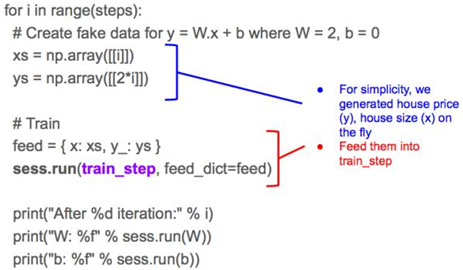你需要将由 x, y_ 所组成的实际数据输入再提供给输入，因为 TensorFlow 将 train_step 分解为它的从属项：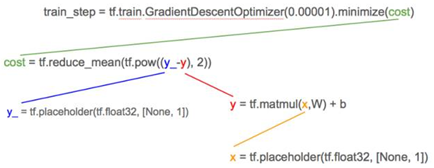从属项的底部是占位符 x，y_；而且正如我们之前提到的，tf.placeholders 是用来表示所要提供的实际数据点值房价 (y_) 和房子面积 (x) 的位置。 结果 循环中的 print 语句将显示 TensorFlow 如何在每次迭代中学习 W 和 b 的「good」值。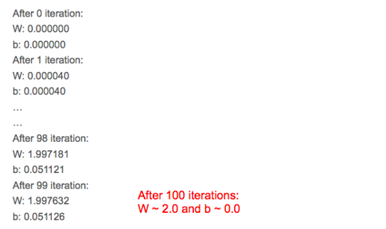小结 我们已经以最简单的形式学习了机器学习；从一个单一特征预测结果。（为简单起见）我们选择了一个线性模型来拟合我们的数据点，定义一个成本函数来表示最佳拟合，并通过反复调整其梯度变量 W 与位置变量 b 来训练我们的模型，使成本函数降到最小。 第二部分 关键点总结及代码 简单回顾 在上一部分，我们使用 TensorFlow 构建并学习了一个带有单一特征的线性回归模型——给定一个特征值（房屋面积/平方米），我们可以预测输出（房价/美元）。 下面是一些总结： 1. 我们有一些房屋面积和房价的数据（灰色点） 2. 我们使用线性回归对这些数据进行了建模（红色虚线） 3. 我们通过训练该线性回归模型的 W（权重）和 b（偏置）找到了最小化「成本」（竖直蓝色实线的长度总和，这些蓝线代表了预测和实际输出之间的差异）的「最好」模型 4. 给定任意房屋面积，我们可以使用该线性模型预测房价（带箭头的蓝色虚线）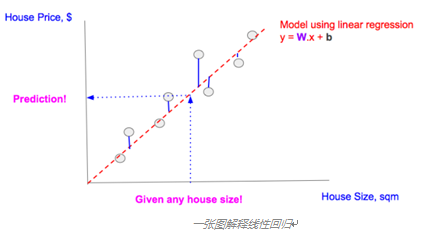在机器学习文献中，我们常常看到「训练（training）」这个词。在这一部分，我们将在 TensorFlow 中理解「训练」的含义。 线性回归建模 Linear Model (in TF notation): y = tf.matmul(x,W) + b 线性回归的目标是寻找 W 和 b，这样对于给定的任意特征值 x，我们可以通过将 W、b 和 x 的值代入到模型中得到预测 y。 但是为了找到能准确做出预测的 W 和 b 的值，我们需要使用可用的数据（许多实际特征 x 和实际输出 y_ 的配对，注意下划线）来「训练」该模型。 解释「训练」 为了找到最佳的 W 和 b 值，我们可以从任意的 W 和 b 值开始。我们也需要定义一个成本函数，该函数可以衡量对于一个给定特征值 x 预测输出 y 和实际输出 y_ 之间差异。为了简单起见，我们使用最简单的最小均方误差（MSE：minimum squared error）作为我们的成本函数。 Cost function (in TF notation): tf.reduce_mean(tf.square(y_ - y)) 通过最小化成本函数，我们可以得到很好的 W 和 b 值。 训练代码实际上非常简单，并且用 [A, B, C, D] 进行了注释，后面我们还会谈到这些代码。完整代码请访问：https://github.com/nethsix/gentle_tensorflow/blob/master/code/linear_regression_one_feature_using_mini_batch_with_tensorboard.py # ... (省略) 变量/常量声明 ... # [A] TensorFlow图 y = tf.matmul(x,W) + b cost = tf.reduce_mean(tf.square(y_-y)) # [B] 用固定「学习率（learn_rate）」训练 learn_rate = 0.1 train_step = tf.train.GradientDescentOptimizer(learn_rate).minimize(cost) for i in range(steps): # [C] 准备数据点 # ... (省略) 准备作为x和y的数据点的代码 ... # [D] 在每个步骤/epoch将数据送入'train_step' feed = { x: xs, y_: ys } sess.run(train_step, feed_dict=feed) 我们的线性模型和成本函数[A]可以表示成下面的 TensorFlow 图：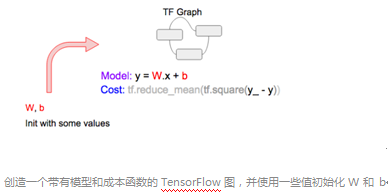接下来，我们选择一个数据点 (x, y_) [C]，然后将其送入[D] TensorFlow 图，从而得到预测 y 和相应的成本。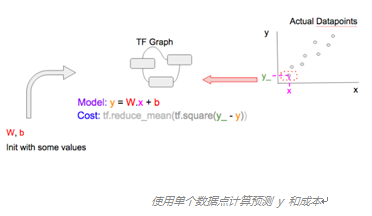为了得到更好的 W 和 b，我们使用TensorFlow 的 tf.train.GradientDescentOptimizer [B]执行梯度下降以降低成本。用非技术的术语来说：给定当前成本，并基于成本岁其它变量（即 W 和 b）的变化方式，优化器（optimizer）将对 W 和 b 执行一些小调整（递增或递减）以使我们的预测更好地契合那个单个数据点。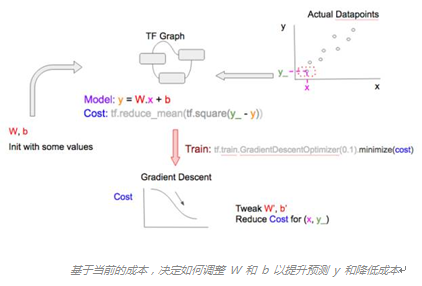训练周期中的最后步骤是在调整 W 和 b 对它们进行更新。注意这里的「周期」用机器学习的术语来说是「epoch」。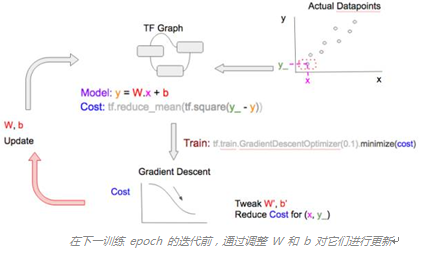在下一训练 epoch 中，重复这些步骤，但使用一个不同的数据点！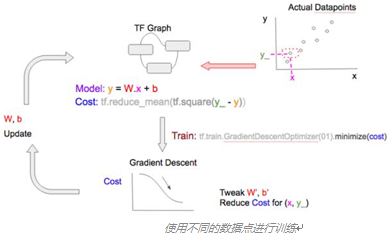使用各种数据点泛化（generalize）我们的模型，即学习可被用于预测任何特征值的 W 和 b 值。注意： • 在大部分情况下，数据点越多，模型的学习和泛化就越好 • 如果你训练的 epoch 比数据点还多，你可以重复使用数据点，这不成问题。梯度下降优化总是会同时使用数据点及其成本（根据该 epoch 的 W 和 b 值从数据点中计算得到）来对 W 和 b 值进行调整；该优化器也许之前已经见过了这个数据点，但成本并不一样，因此它还是可以学到新的东西，并以不同的方式调整 W 和 b 值。 你可以用固定数量的 epoch 训练一个模型，直到其达到令人满意的成本阈值。 训练变量 1.随机、mini-batch、batch 在上面的训练中，我们在每个 epoch 送入单个数据点。这被称为随机梯度下降（stochastic gradient descent）。我们也可以在每个 epoch 送入一堆数据点，这被称为 mini-batch 梯度下降，或者甚至在一个 epoch 一次性送入所有的数据点，这被称为 batch 梯度下降。请看下图的比较，注意这 3 张图的 2 处不同： • 每个 epoch 送入 TensorFlow 图（TF.Graph）的数据点的数量（图右上方） • 梯度下降优化在调整 W 和 b 值时所考虑的数据点的数量（图右下方）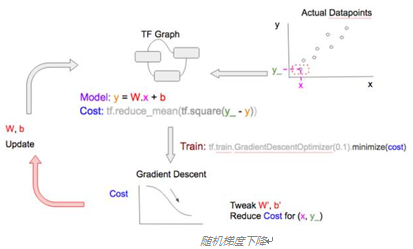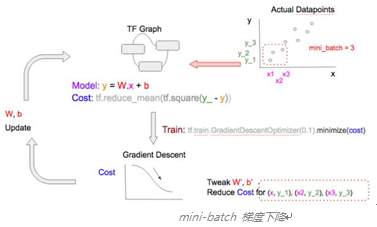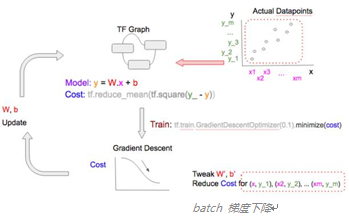每张图中的数据点的数量有 2 个含义。当数据点更多时： • 计算成本和执行梯度下降所需的计算资源（减法、平方、加法）会增加 • 模型的学习和泛化的速度增加 选择随机、mini-batch、batch 梯度下降的优缺点总结在下图中：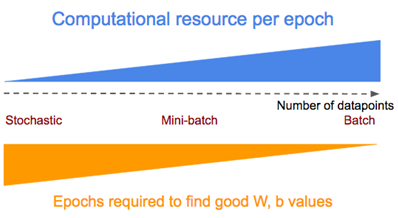要在随机/mini-batch/batch 梯度下降之间切换，我们只需要在将数据点送入训练步骤[D]之前将这些数据点分成不同的 batch 大小，即为 [C] 使用如下的代码片段 # * all_xs: 所有的特征值 # * all_ys: 所有的输出值 # datapoint_size: all_xs/all_ys 中点/项的数量 # batch_size: 配置如下: # 1: 随机模型 # integer < datapoint_size: mini-batch模式 # datapoint_size: batch模式 # i: 当前epoch数量 if datapoint_size == batch_size: # Batch 模式，所以选择所有数据点从 index 0 开始 batch_start_idx = 0 elif datapoint_size < batch_size: # 不可能 raise ValueError(“datapoint_size: %d, must be greater than batch_size: %d” % (datapoint_size, batch_size)) else: # 随机/mini-batch模式: 从所有可能的数据点中分批选择数据点 batch_start_idx = (i * batch_size) % (datapoint_size — batch_size) batch_end_idx = batch_start_idx + batch_size batch_xs = all_xs[batch_start_idx:batch_end_idx] batch_ys = all_ys[batch_start_idx:batch_end_idx] # 将分批的数据点定义为xs, ys, 它们会被送入 'train_step'训练步骤 xs = np.array(batch_xs) ys = np.array(batch_ys) 2.学习率变化 学习率（learn rate）是指梯度下降调整 W 和 b 递增或递减的速度。学习率较小时，处理过程会更慢，但肯定能得到更小成本；而当学习率更大时，我们可以更快地得到最小成本，但有「冲过头」的风险，导致我们没法找到最小成本。 为了克服这一问题，许多机器学习实践者选择开始时使用较大的学习率（假设开始时的成本离最小成本还很远），然后随每个 epoch 而逐渐降低学习率。 TensorFlow 提供了 2 种方法可以做到这一点，详细解释可参考：http://stackoverflow.com/questions/33919948/how-to-set-adaptive-learning-rate-for-gradientdescentoptimizer；但这里进行了总结。 • 使用梯度下降优化的变体 TensorFlow 带有多种支持学习率变化的梯度下降优化器，例如 tf.train.AdagradientOptimizer 和 tf.train.AdamOptimizer. • 使用 tf.placeholder 调整学习率 如同前面所看到的，如果我们在这个例子中声明了 tf.placeholder 来设置学习率，然后在 tf.train.GradientDescentOptimizer 中使用它，我们可以在每个训练 epoch 向其送入一个不同的值，这很像我们给 x 和 y_ 送入不同的数据点，这也是每个 epoch 的 tf.placeholders. 我们需要 2 个小修改： # 修改 [B] ，将 'learn_rate' 设置为'tf.placeholder' # 并将其提供给'learning_rate'参数名tf.train.GradientDescentOptimizer learn_rate = tf.placeholder(tf.float32, shape=[]) train_step = tf.train.GradientDescentOptimizer( learning_rate=learn_rate).minimize(cost) # 修改[D]，包含送入一个'learn_rate'值, # 即 'initial_learn_rate'（初始学习率）除以'i' (当前epoch数) # 注: 这是过于简化的，仅用作示例 feed = { x: xs, y_: ys, learn_rate: initial_learn_rate/i } sess.run(train_step, feed_dict=feed) 小结 我们解释了机器学习中「训练（training）」的含义，以及在 TensorFlow 中通过模型和成本定义、然后循环通过训练步骤（将数据点送入梯度下降优化器）来进行训练的方式。我们还讨论了训练中的常见变量，即改变模型学习时每个 epoch 所用的数据点的大小和改变梯度下降优化器的学习率。 后续内容 • 创建 Tensor Board 来可视化 Tensorflow 的执行，从而检测我们的模型、成本函数或梯度下降中的问题 • 使用多个特征表达线性回归 # linear_regression_one_feature_using_mini_batch_with_tensorboard.py import numpy as np import tensorflow as tf # CUSTOMIZABLE: Collect/Prepare data datapoint_size = 1000 batch_size = 1 steps = 10000 actual_W = 2 actual_b = 10 learn_rate = 0.001 log_file = "/tmp/feature_1_batch_1" # Model linear regression y = Wx + b x = tf.placeholder(tf.float32, [None, 1], name="x") W = tf.Variable(tf.zeros([1,1]), name="W") b = tf.Variable(tf.zeros(), name="b") with tf.name_scope("Wx_b") as scope: product = tf.matmul(x,W) y = product + b # Add summary ops to collect data W_hist = tf.summary.histogram("weights", W) b_hist = tf.summary.histogram("biases", b) y_hist = tf.summary.histogram("y", y) y_ = tf.placeholder(tf.float32, [None, 1], name="y_") # Cost function sum((y_-y)**2) with tf.name_scope("cost") as scope: cost = tf.reduce_mean(tf.square(y_-y)) cost_sum = tf.summary.scalar("cost", cost) # Training using Gradient Descent to minimize cost with tf.name_scope("train") as scope: train_step = tf.train.GradientDescentOptimizer(learn_rate).minimize(cost) all_xs = [] all_ys = [] for i in range(datapoint_size): # Create fake data for y = W.x + b where W = 2, b = actual_b all_xs.append(i%10) all_ys.append(actual_W*(i%10)+actual_b) all_xs = np.transpose([all_xs]) all_ys = np.transpose([all_ys]) sess = tf.Session() # Merge all the summaries and write them out to /tmp/mnist_logs merged = tf.summary.merge_all() writer = tf.summary.FileWriter(log_file, sess.graph_def) init = tf.initialize_all_variables() sess.run(init) for i in range(steps): if datapoint_size == batch_size: batch_start_idx = 0 elif datapoint_size < batch_size: raise ValueError("datapoint_size: %d, must be greater than batch_size: %d" % (datapoint_size, batch_size)) else: batch_start_idx = (i * batch_size) % (datapoint_size - batch_size) batch_end_idx = batch_start_idx + batch_size batch_xs = all_xs[batch_start_idx:batch_end_idx] batch_ys = all_ys[batch_start_idx:batch_end_idx] xs = np.array(batch_xs) ys = np.array(batch_ys) # Record summary data, and the accuracy every 10 steps if i % 10 == 0: all_feed = { x: all_xs, y_: all_ys } result = sess.run(merged, feed_dict=all_feed) writer.add_summary(result, i) else: feed = { x: xs, y_: ys } sess.run(train_step, feed_dict=feed) print("y: %s" % sess.run(y, feed_dict=feed)) print("y_: %s" % ys) print("cost: %f" % sess.run(cost, feed_dict=feed)) print("After %d iteration:" % i) print("W: %f" % sess.run(W)) print("b: %f" % sess.run(b)) # NOTE: W should be close to actual_W, and b should be close to actual_b 第三部分 矩阵和多特征线性回归 快速回顾 之前文章的前提是：给定特征——任何房屋面积（sqm），我们需要预测结果，也就是对应房价（$）。为了做到这一点，我们：

1. 我们找到一条「最拟合」所有数据点的直线（线性回归）。「最拟合」是当线性回归线确保实际数据点（灰色点）和预测值（内插在直线上的灰色点）之间的差异最小，即最小化多个蓝线之和。

2. 使用这条直线，我们可以预测任何房屋的价格。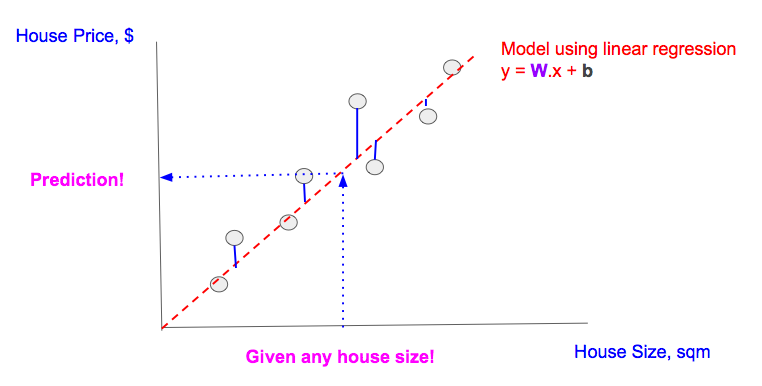多特征线性回归概述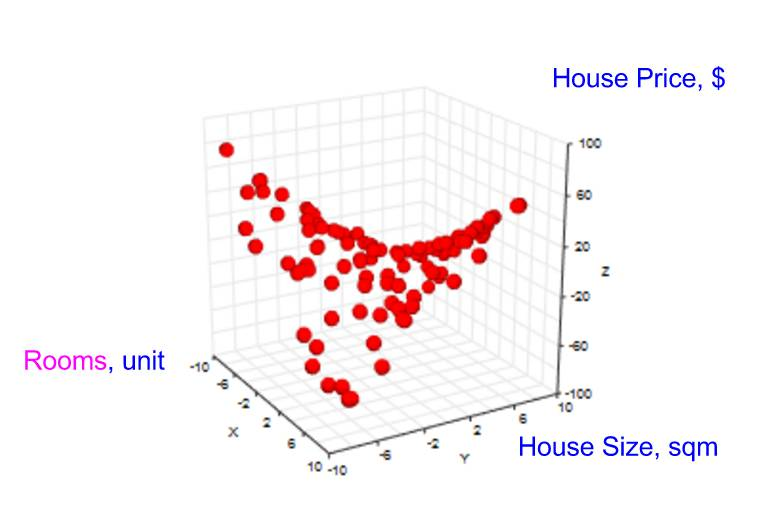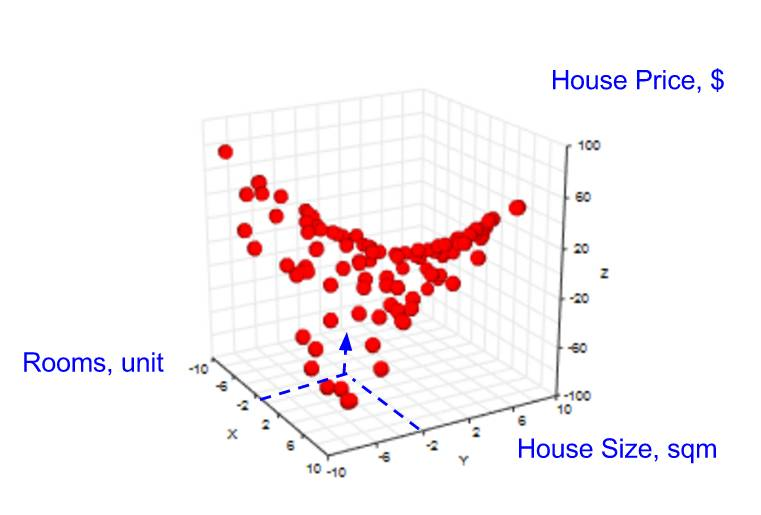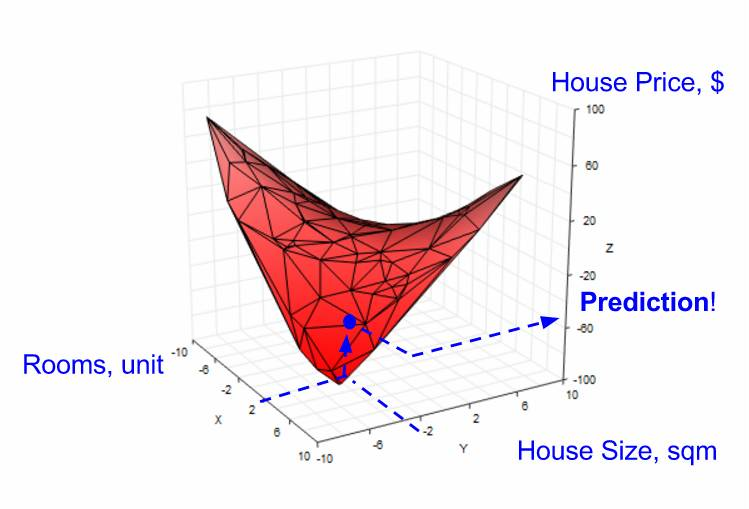多特征线性回归模型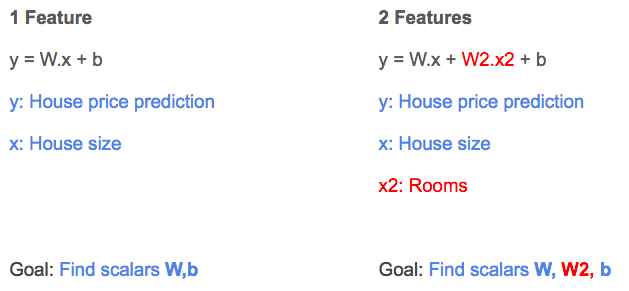Tensorflow 的多特征线性回归

1.快速回顾

• 构建模型（蓝色部分）

• 基于模型构建成本函数（红色部分）

• 使用梯度下降（绿色部分）最小化成本函数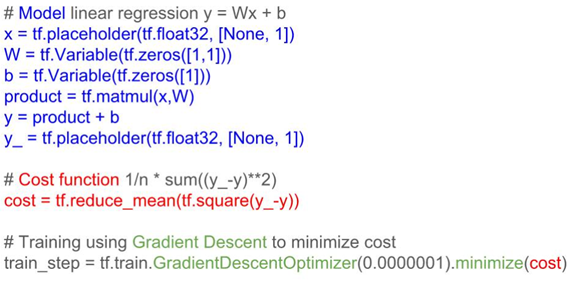2.Tensorflow 的 2 个特征的线性回归

TF 代码中 2 个特征的线性回归方程（如上所述）的变化（相比单特征）用红色显示。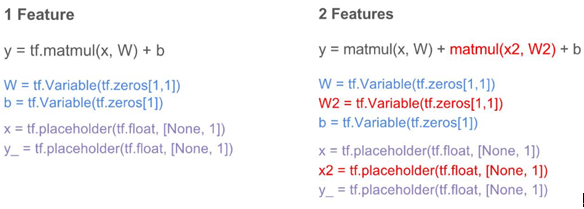解决方法：矩阵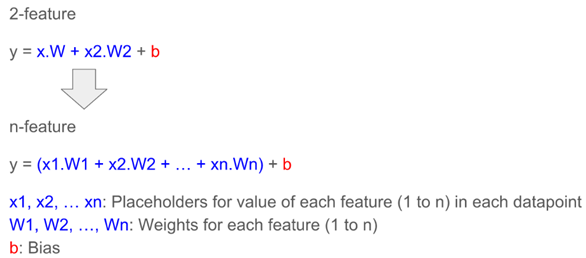• 数据可以用多维表示，这契合我们表征具有 n 个特征的数据点（左下方，也称为特征矩阵）以及具有 n 个权重模型（右下，也称为权重矩阵）的方式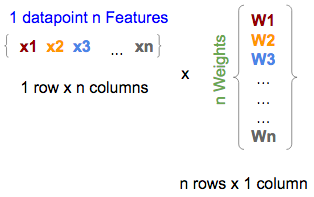x = tf.placeholder（tf.float，[1，n]）
W = tf.Variable（tf.zeros [n，1]）

• 在数学上，矩阵乘法是向量乘法的加总；因此自然地，特征（中间的一个）和权重（右边的）矩阵之间的矩阵乘法给出（左边的）结果，即等于 n 个特征的线性回归公式的第一部分（如上所述），没有截距项。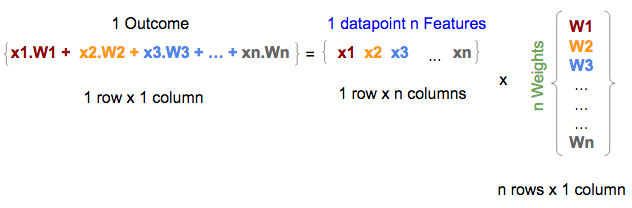• 多行特征矩阵（每行表示数据点的 n 个特征）之间的矩阵乘法返回多行结果，每行代表每个数据点的结果/预测（没有加入截距项）；因此一个矩阵乘法就可以将线性回归公式应用于多个数据点，并对应地产生多个预测（每个数据点对应一个结果）（见下文）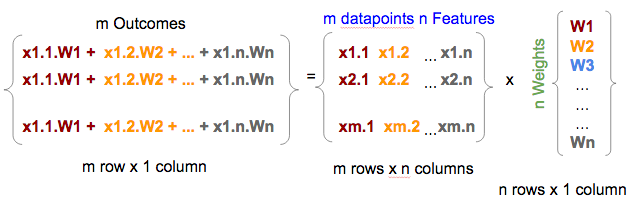x = tf.placeholder（tf.float，[m，n]）
W = tf.Variable（tf.zeros [n，1]）
y = tf.matmul（x，W）
• 最后，向结果矩阵添加常数，也就是将常数添加到矩阵中的每一行

b = tf.Variable(tf.zeros)
y = tf.matmul(x, W) + b

Tensorflow 的多特征备忘单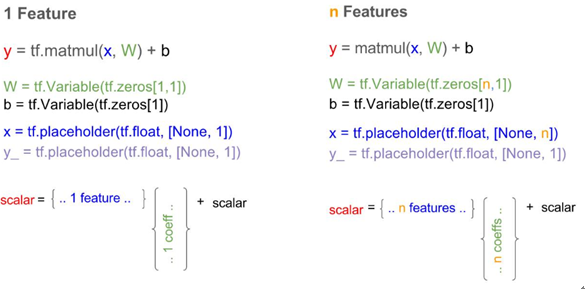Tensorflow 中的单特征与 n 个特征的线性回归模型

第四部分  逻辑回归

逻辑回归的细节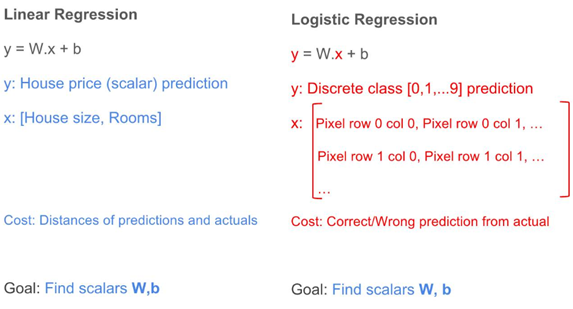• 结果（y）：对于线性回归，结果是一个标量值（可以是任意一个符合实际的数值），例如 50000，23.98 等；对于逻辑回归，结果是一个整数（表示不同类的整数，是离散的），例如 0,1,2，... 9。

• 特征（x）：对于线性回归，特征都表示为一个列向量；对于涉及二维图像的逻辑回归，特征是一个二维矩阵，矩阵的每个元素表示图像的像素值，每个像素值是属于 0 到 255 之间的整数，其中 0 表示黑色，255 表示白色，其他值表示具有某些灰度阴影。

• 成本函数（成本）：对于线性回归，成本函数是表示每个预测值与其预期结果之间的聚合差异的某些函数；对于逻辑回归，是计算每次预测的正确或错误的某些函数。

• 训练：线性回归和逻辑回归的训练目标都是去学习权重（W）和偏置（b）值。

• 结果：线性回归与逻辑回归的目标都是利用学习到的权重和偏置值去预测/分类结果。

协调逻辑回归与线性回归

1.特征变换，x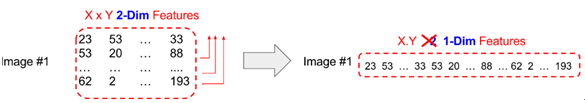2.预测结果转换，y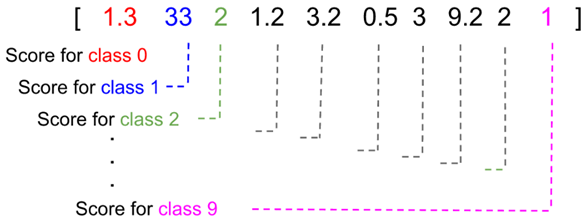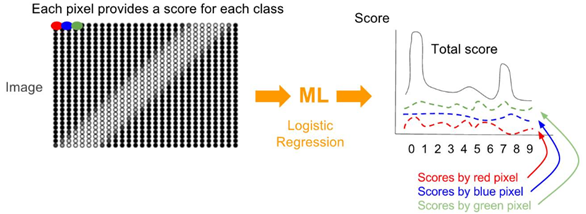3.成本函数的变换

1. 将实际图片的类向量（y'）转化成 one-hot 向量，这是一个概率分布。

2. 将预测类 (y) 转化成概率分布。

3. 使用交叉熵函数去计算成本函数，这表示的是两个概率分布函数之间的差异。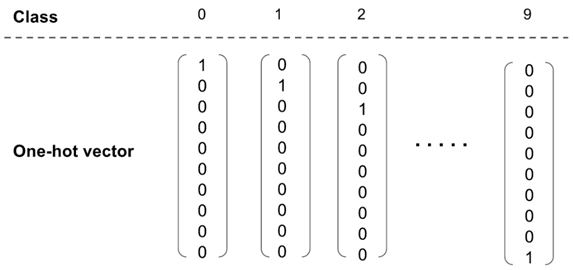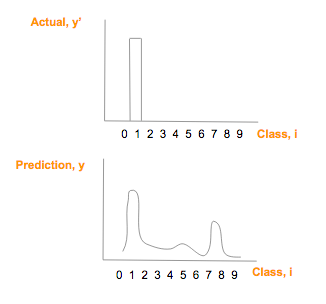• 每个类的概率/分数值在 0-1 之间；

• 所以类的概率/分数和必须是 1；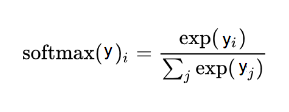softmax 函数，这里 i 是表示 0, 1, 2, …, 9 十类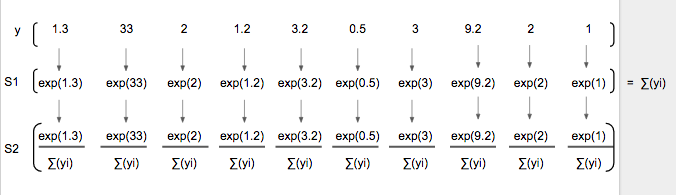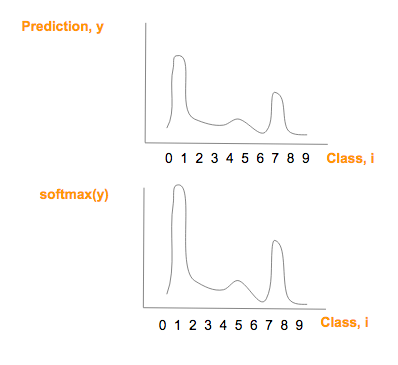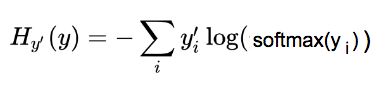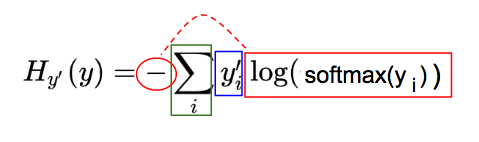• 蓝：实际图像类（y'）对应的 one-hot 图，参看 one-hot 向量部分：

• 红：由预测向量元素（y）经过softmax(y)，-og(softmax(y）一系列变化而来：

• 绿：每一图片类别 i，其中，i = 0, 1, 2, …, 9, 红蓝部分相乘的结果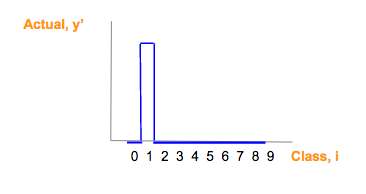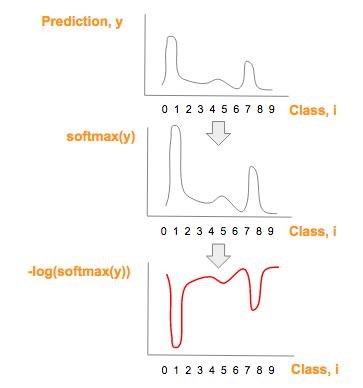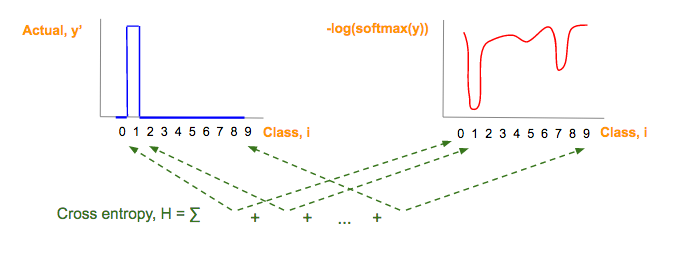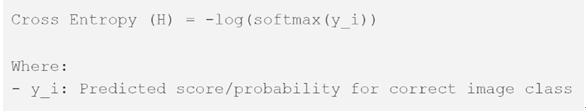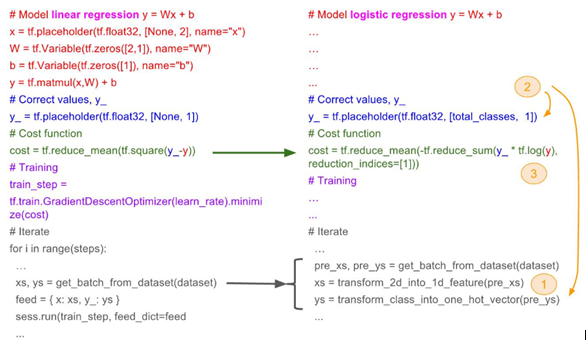1. 将特征变换成一维特征；

2. 将预测结果向量、实际结果向量变化成 one-hot 向量；

3. 将成本函数从平方误差函数变化到交叉熵。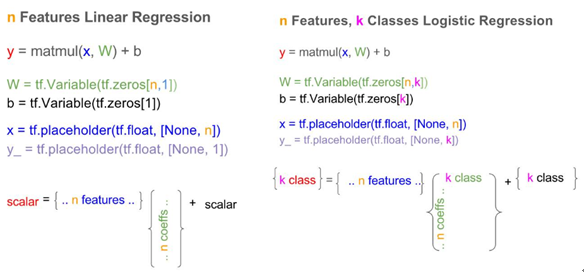总结

01-27

12-23307
10-132382
12-294342
11-20907
05-14409
12-1315万+
02-072775
07-233239
11-23
09-245596
05-111265
04-219160
03-2641万+
12-137万+
12-021429
04-231976¥2 ¥4 ¥6 ¥10 ¥20余额支付 (余额：-- )扫码支付获取中扫码支付点击重新获取扫码支付1.余额是钱包充值的虚拟货币，按照1:1的比例进行支付金额的抵扣。
2.余额无法直接购买下载，可以购买VIP、C币套餐、付费专栏及课程。余额充值